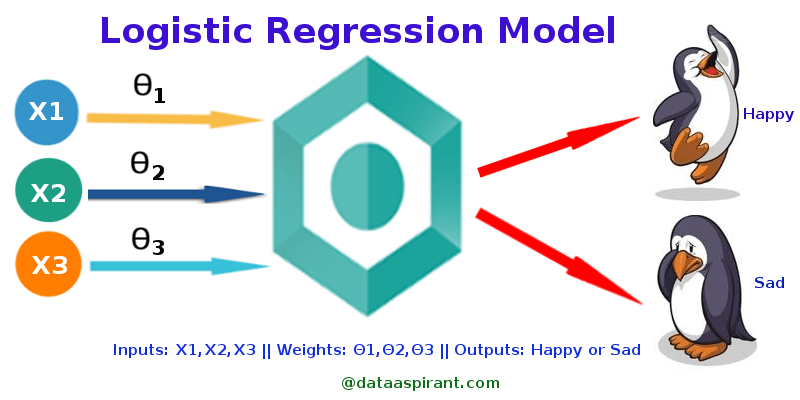Logistic Regression with SciKit-LearnIntroduction

I’ve written articles about Simple Linear Regression and Multiple Linear Regression. Another type of regression analysis is called a logistic regression. Like all simple and multiple regression analysis, logistic regression is also a predictive analysis. The only difference is that while simple and multiple regression returns a quantitative response, logistic regression returns a binary response (success/failure, yes/no, 1/0).

We will model the success probability as p = P(response = 1). The value p will depend on a quantitative predictor p = P(response = 1), so p = p(X). Logistic regression is modeled by the sigmoid curve; and while there are many solutions for the problem, the most common solution is the logit function. Since p(X) will return the probability of success, we will set p(x) to the logit function.

In this article, I’m going to use SciKit-Learn to perform the regression analysis on a problem. The problem I’m attempting to solve was an example given by my Professor at San Jose State University.

Problem

The Challenger disaster in January 1986 was caused by the failure of an O-ring. The incidence could have been prevented because data on failure of these O-rings (as a function outside air temperature) was available at the time of the shuttle launch.

On the morning of January 28, 1986 the air temperature was about 31°F. Even though this value is outside the range of observed temperatures, use the logit model to predict the probability of O-ring failure for the Challenger flight.

Data

Temperature Success/Failure 0 = success 1 = failure
53.0 1.0
56.0 1.0
57.0 1.0
. .
. .
80.0 0.0
81.0 0.0

X will be our temperature in a 2D array and our response will be our variable y, which is also a 2D array.

X = [[53.0],[56.0],[57.0],[63.0],[66.0],[67.0],[67.0],[67.0],[68.0],[69.0],[70.0],[70.0],[70.0],[70.0],[72.0],[73.0],[75.0],[75.0],[76.0],[76.0],[78.0],[79.0],[80.0],[81.0]]
y = [[1.0],[1.0],[1.0],[0.0],[0.0],[0.0],[0.0],[0.0],[0.0],[0.0],[0.0],[1.0],[0.0],[1.0],[0.0],[0.0],[0.0],[1.0],[0.0],[0.0],[0.0],[0.0],[0.0],[0.0]]

SciKit-Learn

Import our necessary Python packages. In this case, we only need SciKit-Learn. From SKLearn, we want to import LogisticRegression()

from sklearn.linear_model import LogisticRegression

Next, we want to create a logistic regression model with 2 parameters: C and solver.

Solver: According to the documentation, the solver parameter specifies the type of optimization algorithm. An optimization algorithm in mathematics is an iterative procedure that tries to find the best solution. In this example problem, we will use the Broyden–Fletcher–Goldfarb–Shanno algorithm. If you want to learn more about this algorithm, refer to this wikipedia page

C: Allows us to specify the strength of the regularization. In other words, the higher the number, the closer the algorithm will try to get to the right prediction. However, setting a too-high of a number would cause your model to overfit. Too small would not reach the optimal solution. In this example, we'll try 25.

model = LogisticRegression(C=25, solver='lbfgs')

Next, we want to fit our data to the model. We do this by simply using the fit() function.

model.fit(X,y)

Run the Model

After running your code, we can grab the coefficients from our logistic regression model. We do this by using the coef_ and intercept_ functions.

m = model.coef_
b = model.intercept_
print(b,m)
Output
intercept_ = [11.74238757] coef_ = [[-0.18837235]]

Interpretation

Based on the model, we can interpret that as temperature increases by one unit, the odds ration will change by a factor of e**m. In this case, m = -0.18837235

The question, what is the probability of failure when the temperature outside is 31°F. Simply let p(31) = ? where X as our predictor equal to 31.

model.predict_proba([])
Output
0.99727578

That means, there is a 99.7% chance of failure if the temperature outside is at 31°F. With that being said, it is no surprise that the challenger exploded in mid-flight. It would be surprising if it did not explode.

Final Thoughts

This example covered logistic regression with one predictor. SciKit-Learn is capable of performing logistic regression with more than one predictor. The only difference we need to change is the solver, C, and the multi_class parameters.